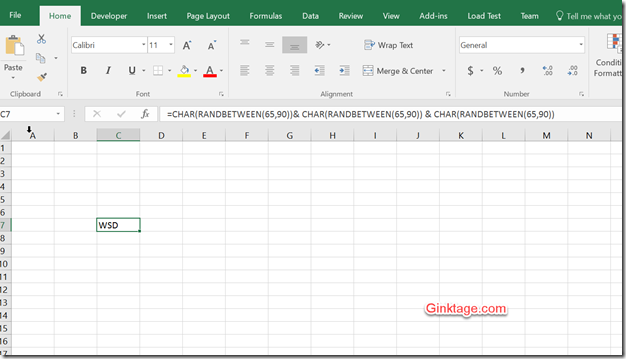# How to generate random strings in Excel?

In one of the previous blog posts , we demonstrated how you can use the function RANDBETWEEN in Microsoft Excel to generate random number in Excel. In this blog post, let’s have a look at generating random strings in Excel.

# How to generate random strings in Excel ?

You can use the combination of the CHAR function and the RANDBETWEEN function to generate the random letter. For example  =CHAR(RANDBETWEEN(65,90)) would generate the random character. The values 65 and 90 represent the ASCII values for the letters A and Z respectively.

Incase you need a string with 3 characters , the formula would be like this.

=CHAR(RANDBETWEEN(65,90))& CHAR(RANDBETWEEN(65,90)) & CHAR(RANDBETWEEN(65,90))